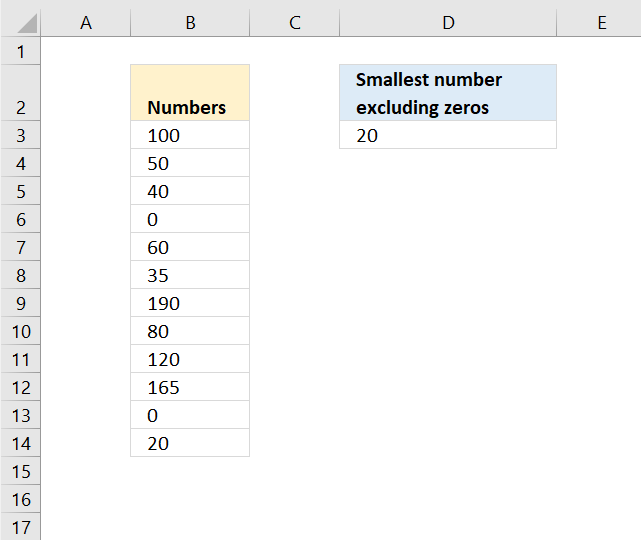Author: Oscar Cronquist Article last updated on November 12, 2018Column B contains numbers, the formula in cell D3 calculates the smallest value excluding zeros. Note that all numbers are either 0 (zero) or larger.

Formula in cell D3:

=MINIFS(B3:B14,B3:B14,"<>0")

The MINIFS function appeared first in Excel 2016 and is a fairly new function. Here is the Excel function syntax:

MINIFS(min_rangecriteria_range1criteria1, [criteria_range2criteria2], ...)

We only have one condition so the function syntax becomes:

MINIFS(min_rangecriteria_rangecriteria)

The min_range argument points to the cells containing the numbers B3:B14.

The criteria_range is the cell range to be evaluated B3:B14.

criteria is the condition, "<>0". <> means not equal to.

If cell in B3:B14 is not equal to 0 (zero) then return cell in B3:B14 and lastly find the smallest value.Use the following array formula if you don't have access to the MINIFS function.

=MIN(IF(B3:B14<>0,B3:B14))

To enter an array formula, type the formula in a cell then press and hold CTRL + SHIFT simultaneously, now press Enter once. Release all keys.

The formula bar now shows the formula with a beginning and ending curly bracket telling you that you entered the formula successfully. Don't enter the curly brackets yourself.

### Explaining formula in cell D3

#### Step 1 - Build logical expression

The IF function requires a logical expression in the first argument in order to return a given value when the logical expression evaluates to TRUE and another value when FALSE.

becomes

{100; 50; 40; 0; 60; 35; 190; 80; 120; 165; 0; 20}<>0

and returns

{TRUE; TRUE; TRUE; FALSE; TRUE; TRUE; TRUE; TRUE; TRUE; TRUE; FALSE; TRUE}.

I have entered the array in column A, note that boolean value FALSE corresponds to cells containing 0 (zero).

#### Step 2 - Filter values in array

The picture shows the array calculated by the IF function, each 0 (zero) is replaced with boolean value FALSE.

becomes

IF({TRUE; TRUE; TRUE; FALSE; TRUE; TRUE; TRUE; TRUE; TRUE; TRUE; FALSE; TRUE}, B3:B14)

becomes

IF({TRUE; TRUE; TRUE; FALSE; TRUE; TRUE; TRUE; TRUE; TRUE; TRUE; FALSE; TRUE}, {100; 50; 40; 0; 60; 35; 190; 80; 120; 165; 0; 20})

and returns

{100; 50; 40; FALSE; 60; 35; 190; 80; 120; 165; FALSE; 20}

#### Step 3 - Find smallest value

The MIN function ignores text, blank and boolean values.

MIN(IF(B3:B14<>0,B3:B14))

becomes

MIN({100; 50; 40; FALSE; 60; 35; 190; 80; 120; 165; FALSE; 20})

and returns 20 in cell D3.